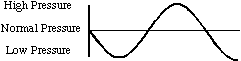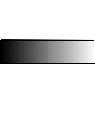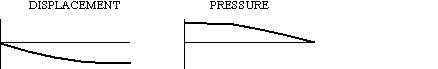Back to "Answer-This" Listing "Answer This" Questions Chapter 3 3-B. Boundary conditions in a tube of air. The figure represents a pressure standing wave in an air tube: What boundary conditions are necessary at the ends of the tube to form this wave?Answer: The pressure is normal at the left end and has an antinode at the right end, which means the left end is open and the right end is closed.   ___________________________ 3-D. Pressure and displacement wave graphs. An air column with one end open and one end closed is vibrating in its first mode. At a particular instant in time, the air molecules are distributed as in the figure. Draw the displacement and pressure wave graphs corresponding to this instant in time.Answer: There is a compression at the left end and normal pressure at the right end. No other pressure nodes or antinodes occur. The displacement must be uniformly towards to left end to form the compression; that is negative displacement. The displacement is maximum to the right at the open end. The graphs that show these features are as follows:Back to "Answer-This" Listing     © William J. Mullin